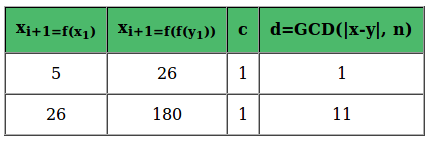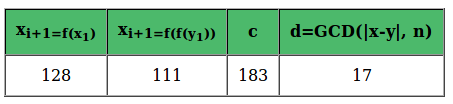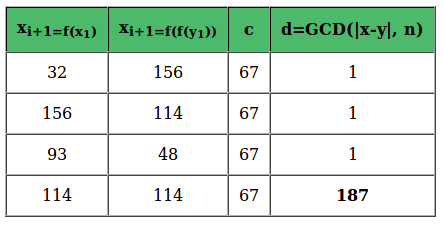# Pollard’s Rho Algorithm for Prime Factorization

• Difficulty Level : Hard
• Last Updated : 06 Apr, 2021

Given a positive integer n, and that it is composite, find a divisor of it.
Example:

```Input: n = 12;
Output: 2 [OR 3 OR 4]

Input: n = 187;
Output: 11 [OR 17]```

Brute approach: Test all integers less than n until a divisor is found.
Improvisation: Test all integers less than √n
A large enough number will still mean a great deal of work. Pollard’s Rho is a prime factorization algorithm, particularly fast for a large composite number with small prime factors. The Rho algorithm’s most remarkable success was the factorization of eighth Fermat number: 1238926361552897 * 93461639715357977769163558199606896584051237541638188580280321.
The Rho algorithm was a good choice because the first prime factor is much smaller than the other one.
Concepts used in Pollard’s Rho Algorithm:

Attention reader! Don’t stop learning now. Get hold of all the important DSA concepts with the DSA Self Paced Course at a student-friendly price and become industry ready.  To complete your preparation from learning a language to DS Algo and many more,  please refer Complete Interview Preparation Course.

In case you wish to attend live classes with experts, please refer DSA Live Classes for Working Professionals and Competitive Programming Live for Students.

1. Two numbers x and y are said to be congruent modulo n (x = y modulo n) if
1. their absolute difference is an integer multiple of n, OR,
2. each of them leaves the same remainder when divided by n.
2. The Greatest Common Divisor is the largest number which divides evenly into each of the original numbers.
3. Birthday Paradox: The probability of two persons having same birthday is unexpectedly high even for small set of people.
4. Floyd’s cycle-finding algorithm: If tortoise and hare start at same point and move in a cycle such that speed of hare is twice the speed of tortoise, then they must meet at some point.

Algorithm

1. Start with random x and c. Take y equal to x and f(x) = x2 + c.
2. While a divisor isn’t obtained
1. Update x to f(x) (modulo n) [Tortoise Move]
2. Update y to f(f(y)) (modulo n) [Hare Move]
3. Calculate GCD of |x-y| and n
4. If GCD is not unity
1. If GCD is n, repeat from step 2 with another set of x, y and c
2. Else GCD is our answer

Illustration:
Let us suppose n = 187 and consider different cases for different random values.

1. An Example of random values such that algorithm finds result
y = x = 2 and c = 1, Hence, our f(x) = x2 + 1.1. An Example of random values such that algorithm finds result faster
y = x = 110 and ‘c’ = 183. Hence, our f(x) = x2 + 183.1.
2. An Example of random values such that algorithm doesn’t find result
x = y = 147 and c = 67. Hence, our f(x) = x2 + 67.Below is C/C++ implementation of above algorithm:

## C++

 `/* C++ program to find a prime factor of composite using``   ``Pollard's Rho algorithm */``#include``using` `namespace` `std;` `/* Function to calculate (base^exponent)%modulus */``long` `long` `int` `modular_pow(``long` `long` `int` `base, ``int` `exponent,``                          ``long` `long` `int` `modulus)``{``    ``/* initialize result */``    ``long` `long` `int` `result = 1;` `    ``while` `(exponent > 0)``    ``{``        ``/* if y is odd, multiply base with result */``        ``if` `(exponent & 1)``            ``result = (result * base) % modulus;` `        ``/* exponent = exponent/2 */``        ``exponent = exponent >> 1;` `        ``/* base = base * base */``        ``base = (base * base) % modulus;``    ``}``    ``return` `result;``}` `/* method to return prime divisor for n */``long` `long` `int` `PollardRho(``long` `long` `int` `n)``{``    ``/* initialize random seed */``    ``srand` `(``time``(NULL));` `    ``/* no prime divisor for 1 */``    ``if` `(n==1) ``return` `n;` `    ``/* even number means one of the divisors is 2 */``    ``if` `(n % 2 == 0) ``return` `2;` `    ``/* we will pick from the range [2, N) */``    ``long` `long` `int` `x = (``rand``()%(n-2))+2;``    ``long` `long` `int` `y = x;` `    ``/* the constant in f(x).``     ``* Algorithm can be re-run with a different c``     ``* if it throws failure for a composite. */``    ``long` `long` `int` `c = (``rand``()%(n-1))+1;` `    ``/* Initialize candidate divisor (or result) */``    ``long` `long` `int` `d = 1; ` `    ``/* until the prime factor isn't obtained.``       ``If n is prime, return n */``    ``while` `(d==1)``    ``{``        ``/* Tortoise Move: x(i+1) = f(x(i)) */``        ``x = (modular_pow(x, 2, n) + c + n)%n;` `        ``/* Hare Move: y(i+1) = f(f(y(i))) */``        ``y = (modular_pow(y, 2, n) + c + n)%n;``        ``y = (modular_pow(y, 2, n) + c + n)%n;` `        ``/* check gcd of |x-y| and n */``        ``d = __gcd(``abs``(x-y), n);` `        ``/* retry if the algorithm fails to find prime factor``         ``* with chosen x and c */``        ``if` `(d==n) ``return` `PollardRho(n);``    ``}` `    ``return` `d;``}` `/* driver function */``int` `main()``{``    ``long` `long` `int` `n = 10967535067;``    ``printf``(``"One of the divisors for %lld is %lld."``,``          ``n, PollardRho(n));``    ``return` `0;``}`

## Java

 `/* Java program to find a prime factor of composite using``   ``Pollard's Rho algorithm */``import` `java.util.*;` `class` `GFG{` `/* Function to calculate (base^exponent)%modulus */``static` `long`  `modular_pow(``long`  `base, ``int` `exponent,``                          ``long`  `modulus)``{``    ``/* initialize result */``    ``long`  `result = ``1``;` `    ``while` `(exponent > ``0``)``    ``{``        ``/* if y is odd, multiply base with result */``        ``if` `(exponent % ``2` `== ``1``)``            ``result = (result * base) % modulus;` `        ``/* exponent = exponent/2 */``        ``exponent = exponent >> ``1``;` `        ``/* base = base * base */``        ``base = (base * base) % modulus;``    ``}``    ``return` `result;``}` `/* method to return prime divisor for n */``static` `long`  `PollardRho(``long`  `n)``{``    ``/* initialize random seed */``    ``Random rand = ``new` `Random();` `    ``/* no prime divisor for 1 */``    ``if` `(n == ``1``) ``return` `n;` `    ``/* even number means one of the divisors is 2 */``    ``if` `(n % ``2` `== ``0``) ``return` `2``;` `    ``/* we will pick from the range [2, N) */``    ``long`  `x = (``long``)(rand.nextLong() % (n - ``2``)) + ``2``;``    ``long`  `y = x;` `    ``/* the constant in f(x).``     ``* Algorithm can be re-run with a different c``     ``* if it throws failure for a composite. */``    ``long`  `c = (``long``)(rand.nextLong()) % (n - ``1``) + ``1``;` `    ``/* Initialize candidate divisor (or result) */``    ``long`  `d = 1L; ` `    ``/* until the prime factor isn't obtained.``       ``If n is prime, return n */``    ``while` `(d == ``1``)``    ``{``        ``/* Tortoise Move: x(i+1) = f(x(i)) */``        ``x = (modular_pow(x, ``2``, n) + c + n) % n;` `        ``/* Hare Move: y(i+1) = f(f(y(i))) */``        ``y = (modular_pow(y, ``2``, n) + c + n) % n;``        ``y = (modular_pow(y, ``2``, n) + c + n) % n;` `        ``/* check gcd of |x-y| and n */``        ``d = __gcd(Math.abs(x - y), n);` `        ``/* retry if the algorithm fails to find prime factor``         ``* with chosen x and c */``        ``if` `(d == n) ``return` `PollardRho(n);``    ``}` `    ``return` `d;``}``  ` `// Recursive function to return gcd of a and b ``static` `long` `__gcd(``long` `a, ``long` `b) ``{ `` ``return` `b == ``0``? a:__gcd(b, a % b);    ``}` `/* driver function */``public` `static` `void` `main(String[] args)``{``    ``long` `n = 10967535067L;``    ``System.out.printf(``"One of the divisors for "` `+ n + ``" is "` `+``          ``PollardRho(n));``}``}` `// This code contributed by aashish1995`

## Python3

 `# Python 3 program to find a prime factor of composite using``# Pollard's Rho algorithm``import` `random``import` `math` `# Function to calculate (base^exponent)%modulus``def` `modular_pow(base, exponent,modulus):` `    ``# initialize result``    ``result ``=` `1` `    ``while` `(exponent > ``0``):``    ` `        ``# if y is odd, multiply base with result``        ``if` `(exponent & ``1``):``            ``result ``=` `(result ``*` `base) ``%` `modulus` `        ``# exponent = exponent/2``        ``exponent ``=` `exponent >> ``1` `        ``# base = base * base``        ``base ``=` `(base ``*` `base) ``%` `modulus``    ` `    ``return` `result` `# method to return prime divisor for n``def` `PollardRho( n):` `    ``# no prime divisor for 1``    ``if` `(n ``=``=` `1``):``        ``return` `n` `    ``# even number means one of the divisors is 2``    ``if` `(n ``%` `2` `=``=` `0``):``        ``return` `2` `    ``# we will pick from the range [2, N)``    ``x ``=` `(random.randint(``0``, ``2``) ``%` `(n ``-` `2``))``    ``y ``=` `x` `    ``# the constant in f(x).``    ``# Algorithm can be re-run with a different c``    ``# if it throws failure for a composite.``    ``c ``=` `(random.randint(``0``, ``1``) ``%` `(n ``-` `1``))` `    ``# Initialize candidate divisor (or result)``    ``d ``=` `1` `    ``# until the prime factor isn't obtained.``    ``# If n is prime, return n``    ``while` `(d ``=``=` `1``):``    ` `        ``# Tortoise Move: x(i+1) = f(x(i))``        ``x ``=` `(modular_pow(x, ``2``, n) ``+` `c ``+` `n)``%``n` `        ``# Hare Move: y(i+1) = f(f(y(i)))``        ``y ``=` `(modular_pow(y, ``2``, n) ``+` `c ``+` `n)``%``n``        ``y ``=` `(modular_pow(y, ``2``, n) ``+` `c ``+` `n)``%``n` `        ``# check gcd of |x-y| and n``        ``d ``=` `math.gcd(``abs``(x ``-` `y), n)` `        ``# retry if the algorithm fails to find prime factor``        ``# with chosen x and c``        ``if` `(d ``=``=` `n):``            ``return` `PollardRho(n)``    ` `    ``return` `d` `# Driver function``if` `__name__ ``=``=` `"__main__"``:` `    ``n ``=` `10967535067``    ``print``(``"One of the divisors for"``, n , ``"is "``,PollardRho(n))``    ` `# This code is contributed by chitranayal   `

## C#

 `/* C# program to find a prime factor of composite using``   ``Pollard's Rho algorithm */``using` `System;``class` `GFG``{` `/* Function to calculate (base^exponent)%modulus */``static` `long`  `modular_pow(``long`  `_base, ``int` `exponent,``                          ``long`  `modulus)``{``  ` `    ``/* initialize result */``    ``long`  `result = 1;` `    ``while` `(exponent > 0)``    ``{``      ` `        ``/* if y is odd, multiply base with result */``        ``if` `(exponent % 2 == 1)``            ``result = (result * _base) % modulus;` `        ``/* exponent = exponent/2 */``        ``exponent = exponent >> 1;` `        ``/* base = base * base */``       ``_base = (_base * _base) % modulus;``    ``}``    ``return` `result;``}` `/* method to return prime divisor for n */``static` `long`  `PollardRho(``long`  `n)``{``  ` `    ``/* initialize random seed */``    ``Random rand = ``new` `Random();` `    ``/* no prime divisor for 1 */``    ``if` `(n == 1) ``return` `n;` `    ``/* even number means one of the divisors is 2 */``    ``if` `(n % 2 == 0) ``return` `2;` `    ``/* we will pick from the range [2, N) */``    ``long`  `x = (``long``)(rand.Next(0, -(``int``)n + 1));``    ``long`  `y = x;` `    ``/* the constant in f(x).``     ``* Algorithm can be re-run with a different c``     ``* if it throws failure for a composite. */``    ``long`  `c = (``long``)(rand.Next(1, -(``int``)n));` `    ``/* Initialize candidate divisor (or result) */``    ``long`  `d = 1L; ` `    ``/* until the prime factor isn't obtained.``       ``If n is prime, return n */``    ``while` `(d == 1)``    ``{``      ` `        ``/* Tortoise Move: x(i+1) = f(x(i)) */``        ``x = (modular_pow(x, 2, n) + c + n) % n;` `        ``/* Hare Move: y(i+1) = f(f(y(i))) */``        ``y = (modular_pow(y, 2, n) + c + n) % n;``        ``y = (modular_pow(y, 2, n) + c + n) % n;` `        ``/* check gcd of |x-y| and n */``        ``d = __gcd(Math.Abs(x - y), n);` `        ``/* retry if the algorithm fails to find prime factor``         ``* with chosen x and c */``        ``if` `(d == n) ``return` `PollardRho(n);``    ``}` `    ``return` `d;``}``  ` `// Recursive function to return gcd of a and b ``static` `long` `__gcd(``long` `a, ``long` `b) ``{ ``  ``return` `b == 0 ? a:__gcd(b, a % b);    ``}` `/* Driver code */``public` `static` `void` `Main(String[] args)``{``    ``long` `n = 10967535067L;``    ``Console.Write(``"One of the divisors for "` `+ n + ``" is "` `+``          ``PollardRho(n));``}``}` `// This code is contributed by aashish1995`

## Javascript

 ``

Output:

`One of the divisors for 10967535067 is 104729`

How does this work?
Let n be a composite (non-prime). Since n is composite, it has a non trivial factor f < n. In fact, there is at least one f <= √n
Now suppose we have to pick two numbers x and y from the range [0, n-1]. The only time we get x = y modulo n is when x and y are identical. However, since f < √n, there is a good chance x = y modulo f even when x and y are not identical (Birthday Paradox).
We begin by randomly selecting x with replacement from the set {0, 1, …, n-1} to form a sequence s1, s2, s3 … Defining &sacute;i = si mod f, our sequence now has each &sacute;i belonging to {0, 1, …, f-1}. Because both the sets are finite, eventually there will be a repeated integer in both. We expect to achieve the repeat earlier in &sacute;i, since f < n.

Now, say &sacute;i = &sacute;j for i ≠ j, then, si = sj modulo d. And hence, |si – sj| will be a multiple of f. As per assumed above, n is also a multiple of f. Together this means that GCD of |si – sj| and n will be positive integral multiple of f, and also our candidate divisor d! The catch here is that we just knew there had to be some divisor of n, and we didn’t even care of its value. Once we hit si and sj (our final x and y) then each element in the sequence starting with si will be congruent modulo f to the corresponding element in the sequence starting with sj, and hence, a cycle. If we graph the sequence si, we will observe the shape of Greek letter Rho (ρ).
At the heart of Rho algorithm is picking up random values and evaluating GCDs. To decrease the costly GCD calculations, we can implement the Pollard’s Rho with Floyd’s cycle detection (which can be understood with the tortoise-hare analogy where the tortoise moves through each element one at a time in order, and the hare starts at the same point but moves twice as fast as the tortoise). We shall have some polynomial f(x) for the same, start with random x0, y0 = x0, and compute xi+1 = f(xi) and yi+1 = f(f(yi)). Since we don’t know much about d, a typical choice for the polynomial is f(x) = x2 + c (modulo n) (Yes, ‘c’ is also be chosen randomly).
Note:

1. Algorithm will run indefinitely for prime numbers.
2. The algorithm may not find the factors and return a failure for composite n. In that case, we use a different set of x, y and c and try again.
3. The above algorithm only finds a divisor. To find a prime factor, we may recursively factorize the divisor d, run algorithm for d and n/d. The cycle length is typically of the order √d.

Time Complexity analysis:
The algorithm offers a trade-off between its running time and the probability that it finds a factor. A prime divisor can be achieved with a probability around 0.5, in O(√d) <= O(n1/4) iterations. This is a heuristic claim, and rigorous analysis of the algorithm remains open.